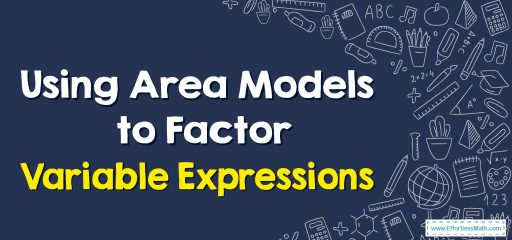# How to Use Area Models to Factor Variable Expressions?

Factors are considered an expression that shows something is multiplied by something else.
Factors would be numbers, terms, variables, or any longer expression.## A step-by-step guide toUsing Area Models to Factor Variable Expressions

The idea of solving multiplication and division problems using the area model comes from the concept of finding the area of a rectangle.
Area of a rectangle$$=$$Length$$×$$Width

An area model is a visual representation of the distributive property of multiplication over addition. To use an area model to factor a variable expression, you can follow these steps:

1. Draw a rectangle with dimensions that match the coefficients and variables in the expression.
2. Identify two smaller rectangles within the larger rectangle that would have dimensions that match the factors you want to find.
3. Write the dimensions of the smaller rectangles as the factors of the original expression.
4. Simplify the expression by using the distributive property, and then simplify further if possible.

Example: To factor x^2 + 6x + 9, you would draw a rectangle with dimensions 1x^2 and 6x, and then identify two smaller rectangles within the larger rectangle that have dimensions (x + 3) and (x + 3), resulting in the factorization (x + 3)^2.

### Using Area Models to Factor Variable Expressions – Example 1

Use the area model for factoring variable expressions.
$$36s+24t$$

Solution:
Step 1: Find the greatest common factor of 36s and 24t. It is 4.
Step 2: Divide each term by the factor. $$36s÷4=9, 24t÷4=6$$
Step 3: Write $$36s+24$$t in factored form. The area model signifies it is equal to the product of 4 and $$9s+6$$t.

### Using Area Models to Factor Variable Expressions – Example 2

Use the area model for factoring variable expressions.
$$18j+12$$

Solution:
Step 1: Find the greatest common factor of 18j and 12. It is 6.
Step 2: Divide each term by the factor. $$18j÷6=3, 12÷6=2$$.
Step 3: Write $$18j+12$$ in factored form. The area model signifies it is equal to the product of 6 and $$3j+2$$.

### What people say about "How to Use Area Models to Factor Variable Expressions? - Effortless Math: We Help Students Learn to LOVE Mathematics"?

No one replied yet.

X
30% OFF

Limited time only!

Save Over 30%

SAVE $5 It was$16.99 now it is \$11.99